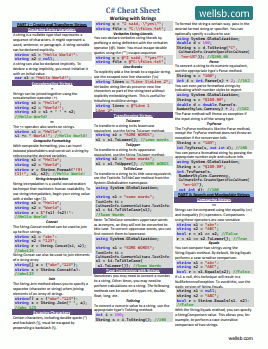# C# Ternary Conditional Operator ?:

## TERNARY (?:) CONDITIONAL OPERATOR IN C#

by

The inline conditional operator, or ternary ( ?: ) operator, in C# is a useful shorthand for replacing an If... Else... statement. In this tutorial, we will learn how and when to use the conditional operator.

In the previous lesson, we learned about using conditional statements to determine which block of code to execute. Consider the following example.

``````int myNumber = 12;
string message = "";

if (myNumber > 10)
message = "greater than 10.";
else
message = "less than or equal to 10.";

Console.WriteLine("The number is " + message);
``````

This code snippet uses an if... else... construct that compares the value of some variable, myNumber, with the number 10. If myNumber is larger than 10, the line "The number is greater than 10." is written in the console. If the number is not larger than 10, the line "The number is less than or equal to 10." is printed to the console.

In this example, myNumber is 12. Since 12 is larger than 10, the first code block will run.

## C# Ternary Shorthand ( ?: ) Example

With the ternary operator, or conditional operator, we can substitute lines 10-15 in the above example with a single line of code. The ternary condition operator ( ?: ) can be used to assign a value to a variable as follows:

``````myVariable = (condition) ? value if true : value if false;
``````

The question mark ( ? ) and the colon ( : ) are the delineating elements of this shorthand notation. If the condition before the question mark is true, myVariable will be assigned the value between the question mark and the colon. If the condition before the question mark is false, myVariable would be assigned the value after the colon. As always, the statement is terminated with a semi-colon ( ; )

``````string message = (myNumber > 10) ? "greater than 10." : "less than or equal to 10.";
``````

In our example, we can replace lines 10-15 using the ternary shorthand notation as shown above. This line of code evaluates whether the variable myNumber is larger than 10. If it is, the variable message is assigned the value "greater than 10." If the condition evaluates to false, the variable message is assigned the value value "less than or equal to 10."

Our complete sample code is below:

``````using System;

namespace Ternary
{
class Program
{
static void Main(string[] args)
{
int myNumber = 12;

string message = (myNumber > 10) ? "greater than 10." : "less than or equal to 10.";

Console.WriteLine("The number is " + message);
}
}
}
``````

## The Bottom Line

In this tutorial, we described the ternary ( ?: ) or conditional operator. You learned how this shorthand notation can be used to substitute simple If... Else... conditional statements in C#. The trick is not useful when we have multiple conditions to evaluate, but for simple if-else conditions, it is a great way to reduce the number of lines in our code. Let me know your questions in the comments below!

#### Don't stop learning!

There is so much to discover about C#. That's why I am making my favorite tips and tricks available for free. Enter your email address below to become a better .NET developer.

#### Did you know?Our beautiful, multi-column C# reference guides contain more than 150 tips and examples to make it even easier to write better code.